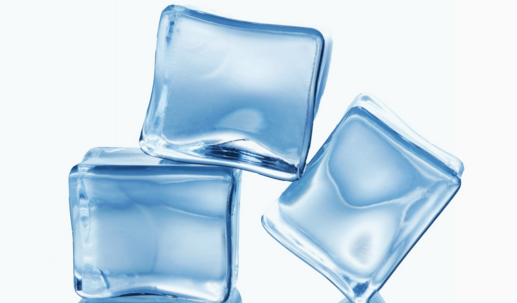It's just square root

Algebra Level 3$\text{ICE}^3=\sqrt{\text{ICE}^6}$

If $\text{ICE}$ is a real number, state the above equation as true/false.

Clarification: $\text{ICE}$ is one variable. In this problem it is not considered as the product of $\text{I,C}$ and $\text{E}$.

×